# Low-Noise Amplifier (LNA) Matching Techniques for Optimizing Noise Figures

### Abstract

An RF amplifier is an active network that increases the amplitude of weak signals, thereby allowing further processing by the receiver. Receiver amplification is distributed between RF and IF stages throughout the system, and an ideal amplifier increases the desired signal amplitude without adding distortion or noise. Unfortunately, amplifiers are known to add noise anddistortion to the desired signal. In a receiver chain, the first amplifier after the antenna contributes most to the system noise figure. Adding gain in front of a noisy network reduces the noise contribution from that network.

### Amplifier Noise Figure

To analyze the effect of circuit noise, one can model the noisy circuit as a noiseless circuit plus external noise sources. For a noisy, two-port network with internal noise sources (Figure 1a), the effects of those sources are represented by the external noise-voltage sources Vn1 and Vn2, placed in series with the input and output terminals, respectively (Figure 1b). Those sources must produce the same noise voltage at the circuit terminals as the internal noise sources. The values of Vn1 and Vn2 are calculated in equations 1 and 2. Representing the noise-free, two-port network in Figure 1b by its Z parameters:and:Equations 1 and 2 show that the Vn1 and Vn2 values can be determined from open-circuit measurements in the noisy two-port network. It follows from these equations that when the input and output terminals are open (I1 = I2 = 0) (equations 3 and 4):and: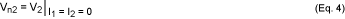In other words, Vn1 and Vn2 equal the corresponding open-circuit voltages.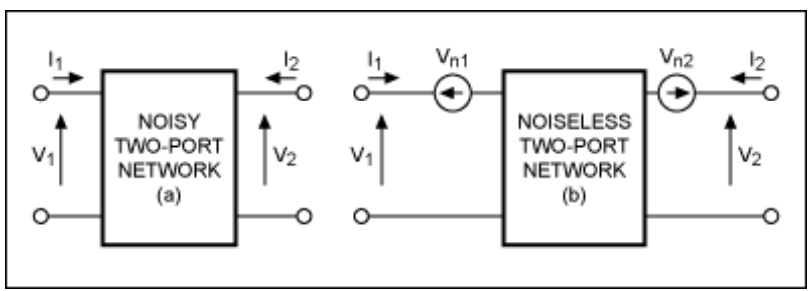In an alternate representation of the noisy two-port network (Figure 2), the external sources are the current noise sources In1 and In2. Equations 5 and 6 represent the noise-free two-port network: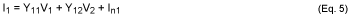and:The values of In1 and In2 in Figure 2 follow from short circuit measurements taken in the noisy two-port network, as shown in equations 7 and 8:and:Other representations, besides those shown in Figures 1b and 2, can be derived for a noisy, two-port network. A convenient representation for noise analysis places the noise source at the input of the network (Figure 3).Representing the noise-free, two-port network in Figure 3 by its ABCD parameters in equations 9 and 10 show:and:Equations 9 and 10 show that there is no simple way to evaluate Vn and In in Figure 3, using open- and short-circuit measurements. From a practical point of view, those values (Vn and In) can be expressed in terms of noise voltages Vn1 and Vn2 in Figure 1b (which require only open-circuit measurements).

The relationship between noise sources Vn and In in Figure 3 and noise sources Vn1 and Vn2 in Figure 1b is derived as follows. Using Z parameters to represent the noise-free, two-port network in Figure 3: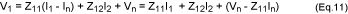and:Comparing equations 1 and 2 with equations 11 and 12, it follows that: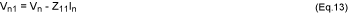and: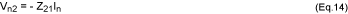Hence, solving equations 13 and 14 for Vn and In gives:and: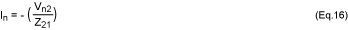An alternate method for determining Vn and In relates them to noise sources In1 and In2 in Figure 2. It is easy to show that the relations in this case are: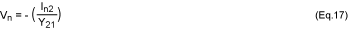and: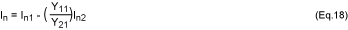A source connected to the noisy two-port network (Figure 4) is represented by a current source with admittance Ys. It is assumed that noise from the source is uncorrelated with noise from the two-port network. Thus, noise power is proportional to the mean square of the short-circuit current (denoted by active-low Isc²) at the input port of the noise-free amplifier; and noise power due to the source alone is proportional to the mean square of the source current (active-low Is²). Hence, the noise figure F is given by: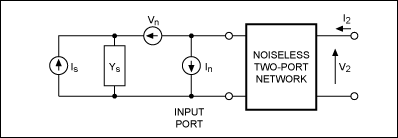Because Isc = -Is + In +VnYs, it follows that the mean square of Isc is given by equation 20:Because noise from the source and noise from the two-port network are uncorrelated: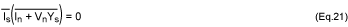and equation 20 reduces to:Substituting equation 20 into equation 19 gives:There is some correlation between external sources Vn and In. Hence, In can be written as the sum of two terms—one uncorrelated to Vn (Inu) and one correlated to Vn (Inc). Thus:Furthermore, the relation between Inc and Vn in terms of a correlation admittance Yc is defined as:Yc is not an actual admittance in the circuit; it is defined by equation 25 and calculated as follows. From equation 24: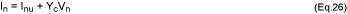Multiplying equation 26 by Vn*, taking the mean, and observing that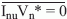: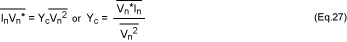Substituting equation 26 into equation 23 produces the following expression for F:Noise produced by the source is related to the source conductance by: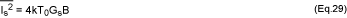where Gs = Re[Ys]. The noise voltage can be expressed in terms of an equivalent noise resistance Rn as:and the uncorrelated noise current can be expressed in terms of an equivalent noise conductance Gu: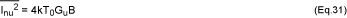Substituting equations 29, 30, and 31 into equation 28, and letting: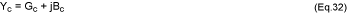and: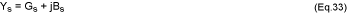gives:The noise factor can be minimized by properly selecting Ys. From equation 34, F is decreased by selecting:Hence, from equation 34:The dependence of the expression in equation 34 on Gs can be minimized by setting: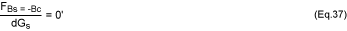This gives: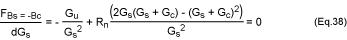Solving for Gs: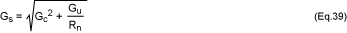The values of Gs and Bs in equations 39 and 35 give the source admittance, which results in the minimum (optimum) noise figure. This optimum value of the source admittance is commonly denoted by Yopt = Gopt + jBopt; that is: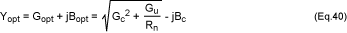From equation 36, the minimum noise figure Fmin is:Solving equation 39 for Gu/Gopt and substituting into equation 41 gives:Using equation 42, equation 34 can be expressed as:Solving equation 39 for Gu and substituting into equation 43, the expression for F can be simplified: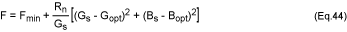Equation 44 shows that F depends on Yopt = Gopt + jBopt, and on Fmin. When these quantities are specified, the value of noise figure F can be determined for any source admittance Ys. This equation can also be expressed as: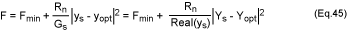where m = Rn/Z0 is the normalized noise resistance and ys = YsZ0 is the normalized source admittance:yopt is the normalized value of the optimum source admittance:Admittances ys and yopt can be expressed in terms of reflection coefficients:Expressing ys and yopt in terms of reflection coefficients helps formulate the noise figure (equation 45) as a function of those coefficients. This formulation is more convenient for industrial LNA applications because in most data sheets, the LNA characteristics are expressed as a table of S parameters and the optimum reflection coefficient Gopt vs. frequency:When the noise figure is expressed as a function of a circle, it can be used with a Smith chart for optimum noise-figure matching in specific applications:For LNA input matching, a noise circle is positioned on the Smith chart as follows:From equations 51 and 52, one can visualize the noise performance of an LNA by plotting the noise circles on the Smith chart. This technique allows the designer to see the effect of tuning in order to estimate the practical noise performance.

### Designing for Optimum Noise Figure

For any two-port network, the noise figure measures the amount of noise added to a signal transmitted through the network. For any practical circuit, the signal-to-noise ratio (SNR) at its output is worse (smaller) than at its input. In most circuit designs, however, the noise contribution of each two-port network can be minimized through a judicious choice of operating point and source resistance.

The preceding section demonstrates that for each LNA (indeed, for any two-port network), there exists an optimum noise figure. LNA manufacturers often specify an optimum source resistance in the data sheet. As an alternative, data sheets for the MAX2656 and other LNAs specify an optimum source-reflection coefficient.

To design an amplifier for minimum noise figure, determine (experimentally or from the data sheet) the source resistance and bias point that produce the minimum noise figure for that device. Then force the actual source impedance to "look like" that optimum value with all stability considerations still applying. If the Rollet stability factor (K) is calculated to be less than 1 (K is defined as a figure of merit for LNA stability), then you must be careful in choosing the source and load-reflection coefficients. For an accurate depiction of the unstable regions, it is best to draw stability circles.

After providing the LNA with optimum source impedance, the next step is to determine the optimum load-reflection coefficient (ΓL) needed to properly terminate the LNA's output:where ΓS is the source-reflection coefficient necessary for minimum noise figure. (The asterisk in the above equation indicates the conjugate of the complex quantity ΓL.)

### Applications

A practical example to illustrate the theory of optimum noise matching for LNAs is the MAX2656 - an LNA (Figure 5) with high third-order adjustable intercept point (IP3). Designed for PCS phone applications with gain selected by logic control (14.5dB in high-gain mode and 0.8dB in low-gain mode), the amplifier exhibits an optimum noise figure of 1.9dB (depending on the value of bias resistor RBIAS). The MAX2655/MAX2656 IP3 is adjusted with a single external bias resistor (RBIAS), which lets you optimize the supply current for specific applications.Figure 5's application employs a MAX2656 LNA operating at a PCS receiver frequency of 1960MHz and noise figure of 2dB (as requested by design). It must operate between 50Ω terminations. As described in the MAX2656 data sheet, the optimum bias resistance (RBIAS) for minimum noise figure is 715Ω. The optimum source-reflection coefficient ΓOPT for minimum noise figure in a 1960MHz application (FMIN = 1.79dB) is:A source impedance with noise-equivalent resistance RN = 43.2336Ω yields the minimum noise figure.

A MAX2656 LNA operating at 1960MHz has the following S parameters (expressed as magnitude/angle):

- S11 = 0.588/-118.67°
- S21 = 4.12/149.05°
- S12 = 0.03/167.86°
- S22 = 0.275/-66.353°

The calculated stability factor (K = 2.684) indicates unconditional stability, so we can proceed with the design. Figure 5 shows design values for the inputmatching network. First, a Smith chart for input matching shows (in blue) the 2dB constant-noise circle requested by design (Figure 6). For comparison, note the dotted-line depiction of constant-noise circles corresponding to the noise figures of 2.5dB, 3dB, and 3.5dB.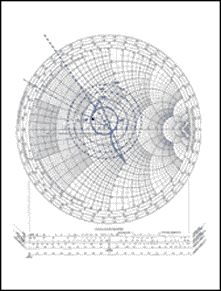For convenience, we chose a source-reflection coefficient of ΓS = 0.3/150° on the 2dB constant-noise circle. The normalized 50Ω source resistance is transformed to ΓS using two components: the arc ΓSA (clockwise in the impedance chart) gives the value of series inductance L1. Arc BO (clockwise in the admittance chart) gives the value of shunt capacitor C1.

The value of arc ΓSA measured on the plot is 0.3 units, so Z = 50 x 0.3 = 15Ω. Thus, L1 = 15/ω = 15/(2πf) = 15/[2π x (1.96 x109)] = 1.218nH, rounded to 1.2nH. The value of the arc BO measured on the plot is 0.9 units, so 1/Y = Z = 50/0.9 = 55.55Ω. Thus, C2 = 1/(55.55 x ω) = 1/(55.55 x 2πf) = 1/[55.55 x 2π x (1.96 x 109)] = 1.46pF, rounded to 1.5pF.

C1 is simply a high-valued DC-isolation capacitor and does not interfere with the input matching. The chosen ΓS provides the load-reflection coefficient needed to properly terminate the LNA:This value and the normalized load-resistance value are plotted in Figure 7, which also shows a possible method for transforming the 50Ω load into ΓL. For this example, note that a single series capacitor provides the necessary impedance transformation.The arc OΓL (counterclockwise in the impedance chart) gives the value for series capacitor C3. The value of arc OΓL measured on the plot is 0.45 units, so Z = 50 x 0.45 = 22.5Ω. Thus, C3 = 1/(22.5 x ω) = 1/(22.5 x 2πf) = 1/[22.5 x 2π x (1.96 x 109)] = 3.608pF, rounded to 3.6pF.

### Conclusion

These calculations have determined the matching components required for optimum noise performance in the LNA of Figure 5. Of course, in low-cost applications where optimum noise performance is not mandatory, C3 can be omitted, and the MAX2656 can be connected directly to a 50Ω system.

### References

1. Gonzalez, Guillermo; Microwave Transistor Amplifiers, Analysis & Design; Second Edition, Prentice Hall, Upper Saddle River, New Jersey 07458.
2. Bowick, Chris; RF Circuit Designs; Howard W. Sams & Co. Inc., a publishing subsidiary of ITT.